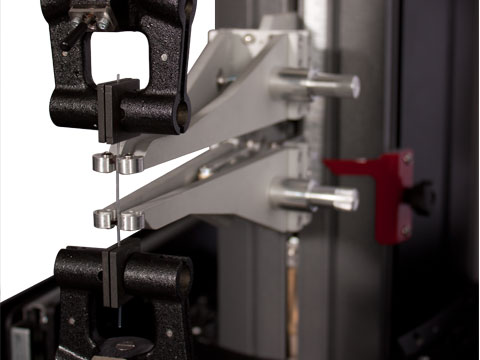Material Testing

Material Testing is a critical process that determines whether a product is reliable, safe, and predictable in function. Material testing is basically divided into two major categories: destructive testing and nondestructive testing.

Destructive testing is defined as a process where a material is subjected to a load in a manner that will ultimately cause the material to fail. Machines have been developed specifically to conduct destructive testing. These machines exert force on the sample and record information such as resulting deformation, the amount of stress that builds up inside the sample, elastic behavior, strength, etc.

When non-destructive testing is performed on a material, the part is not permanently affected by the test. The part is usually still serviceable. The purpose of non-destructive testing is to determine whether the material contains imperfections.

Over many years, tests have been developed for measuring the common properties of engineering materials, including acoustical, electrical, magnetic, physical, optical, and thermal properties. But why is material testing so significant?

K1 – Distinguish between stress, strain, and the modulus of elasticity.

K2 – Distinguish between elastic and plastic deformation.

K3 – Describe the relationship between the tensile force applied to a material and the elongation of the material as it deforms elastically, plastically, and then ruptures. Know the relevant equations.

K4 – Describe strain energy and its significance.

S1 – Calculate minimum or maximum design parameters to ensure a safe or reliable product using material strength properties.
S2 – Measure (or model) axial force and elongation data of material samples and create stress-strain diagrams describing the intrinsic properties of the materials.
S3 – Identify and calculate test sample material properties using a stress-strain curve.

Recommended reading: Structures, or Why Things Don't Fall Down, by J.E. Gordon (Da Capo Press, 1978)

Resources

Start by reading this short overview of stress and strain. While the article focuses on a strain gauge, you're not so much interested in this piece of equipment as you are the concepts surrounding it.

Stress and Strain, Elastic and Plastic Deformation - website (explainthatstuff.com)

Stress and Strain - website (nde-ed.org)

Elastic and Plastic Deformation - website (nde-ed.org)

Modulus of Elasticity - website1 and website2 (wiseGEEK)

Tensile Properties - website (nde-ed.org)

Video #1: An Introduction to Stress and Strain

Video #2: Elastic and Plastic Deformation

Video #3: Strain Energy and Toughness

A tensile test on a piece of rubber.

Tensile testing is routine work for many scientists and materials engineers. Understanding the results of a test requires some expertise, but actually performing a test is quite easy. Simply clamp a "dogbone" sample into the jaws of the machine, input a few values into the software (e.g. TestWorks) running on an attached computer, and press the green "Go" button.

Let's take a look at a few screen shots from an actual test below. The image on the left is the Setup Page; the values in the table are input by the user. The image on the right is the Results Page; the graph shown is the standard stress-strain graph so often encountered in materials testing.

[Click on an image to enlarge it.]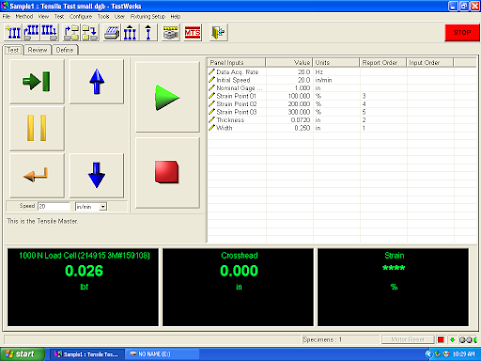Tensile Test Setup Page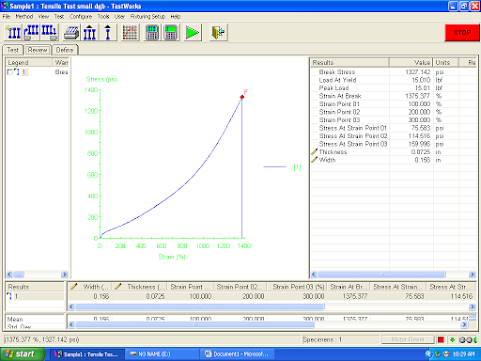Tensile Test Results

Can we make sense of the screen above? Let's discuss each item on the screen to gain some comfort in discussing tensile testing.

Data Acq. Rate - this is the rate at which the computer acquires data, which means reading

values from the attached sensors; in this case, 20.0 times per second (20.0 Hz), which is

normally plenty high

Initial Speed - this is the rate at which the jaws pull apart; 20.0 inches per minute is fast, but

it's appropriate for rubber; we'd use a much smaller speed if we were stretching metal

Nominal Gage - this is the initial distance between the jaws, which is also the length of the  exposed sample; this value is used in calculating strain

Strain Point 01 - this identifies a strain value at which the computer is to return the accompanying    stress; the computer is collecting many ordered points (strain, stress), but we want to highlight this  particular strain (here, 100.000% strain); note: this is a nonstandard input

Strain Point 2 and 3 - same as Strain Point 1

Thickness - this is the thickness of the dogbone sample; we see that it was only 0.072 inches;  note: some dogbones are rectangular in cross-section, like the one used in this test, and some  are circular in cross-section, in which case you might enter the sample's diameter instead of  thickness; the software needs this value to calculate the sample's cross-sectional area, in order to  calculate strain

Width - this is the width of the dogbone sample; it's need to calculate the cross-sectional area of  the sample, for purposes of calculating the strain; you would not input a width for a circular  dogbone (see above)The testing machine is equipped with a 1000 N Load Cell, which is a force sensor able to measure loads up to 1000 newtons. Its current value, according to the image, is 0.026 lbf or pounds of force (which is about 0.12 N). Prior to running the test, this should read 0.000 or at least really close to it. If it doesn't read close to 0, "calibrate" the sensor.

Let's examine [some of] the test results.

Break Stress - the stress was 1327.142 psi when the sample broke in two

Peak Load - the force or load reached a maximum of 15.01 lbf; for this particular sample, this  would have occurred at the break point

Strain at Break - the strain was 1375.377% when the sample broke; this is very high and is only  characteristic of some rubbers; for an alloy of steel, you might have a Break Strain of 20%

Stress at Strain Point 01 - the stress was 75.583 psi when the material reached a strain of  100.000%, which equates to a doubling of length

The Stress vs Strain graph above is characteristic of elastomers (or rubbers). A metal is more likely to have the shape shown below.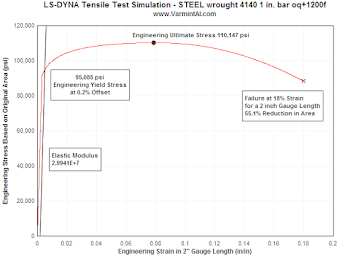Let's examine a data sheet from MatWeb for:

AISI 1010 Steel (cold drawn)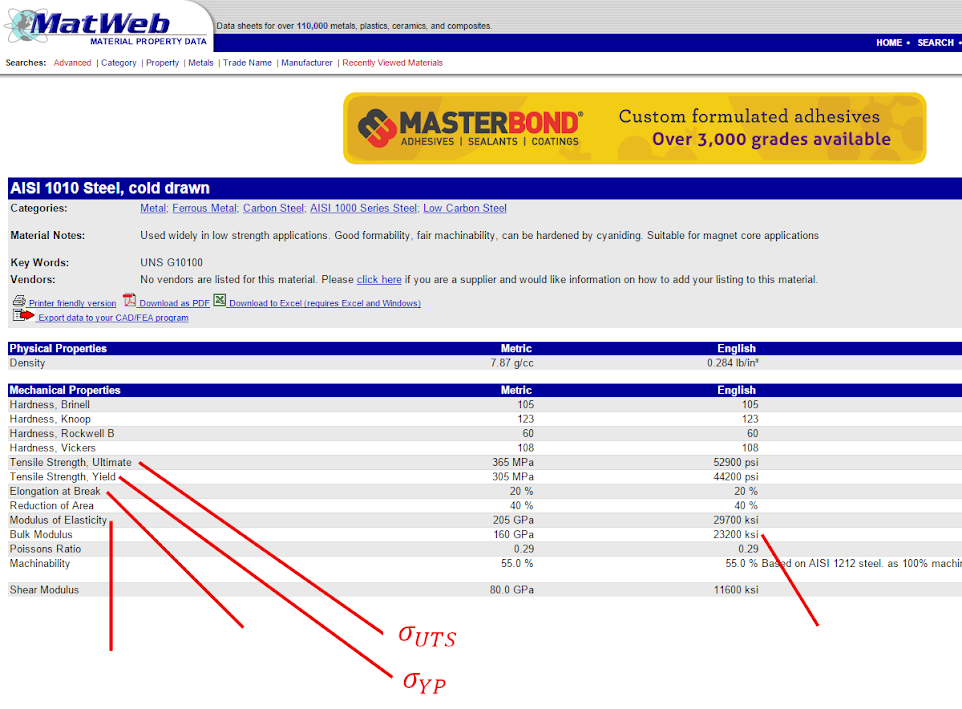the strain, ε

(at break)

E (aka Young's Modulus)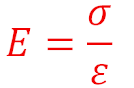kilopounds per square inch

This particular type of steel is low in carbon (which technically means it's 0.55-1.05% carbon). As the % of carbon increases, the steel becomes harder and stronger but also less ductile and more difficult to weld. High carbon steels have up to 2.5% carbon. Of course, most of the steel is iron (Fe). (So what do you call steel with 0% carbon? Yes, iron. Good job.) Very small quantities of other elements can have a significant effect on the properties and quality of the steel, for good or for bad. (If you're wondering why it's called "AISI 1010", check out this Wikipedia page.)

What does it mean for the Modulus of Elasticity to be 29700 ksi?

It takes 29,700,000 pounds to stretch a square inch rod of steel 100%, thus doubling its length. This is impractical, though. For one thing, a decent piece of steel will break before it can be stretched to twice its length. Even if it didn't break, it would not be deforming elastically for most of the stretch, thus invalidating the use of Young's Modulus for calculation purposes. So we ratio down, to get a more practical value. It would take 297,000 psi (1% of 29700 ksi) to stretch the steel rod by 1%.

What else is important to know? Plenty, but let's just look at the Yield Tensile Strength. A stress of 44,200 psi is capable of deforming the steel plastically, which means that when the stress is reduced or removed, the steel doesn't return to its original shape but now has some permanent strain. If this material were to be used in bridge construction, the stress on it should be kept well below 44,200 psi. Actually, the industry standard is to allow a tension stress no more than about 50% of the Yield Tensile Strength, which in this case is 22,100 psi. This is achieved through both sound design and choosing the right type of steel.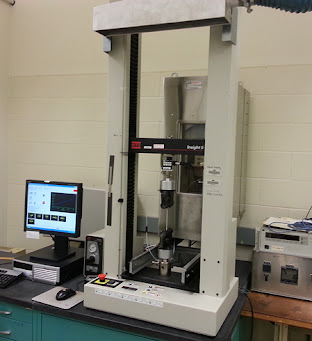Photo: A tensile testing machine. In the same way people refer to a tissue as a Kleenex, people often call this machine an Instron (after a popular maker of testing machines).

Practice Problems involving Stress, Strain, and Modulus of Elasticity - pdf (on Google Drive) - PLTW worksheet

More Practice Problems - pdf (on Google Drive)

And while we're talking about steel...Eighty-three thousand tons of steel were used in the construction of San Francisco's famed Golden Gate Bridge. The steel was shipped to the site from America's East Coast by way of the Panama Canal.

Around 30 painters continuously paint the steel bridge (a bright "International Orange") for the same reason that Mars is red.Image Credit: NASA/JPL/Cornell

You can find a virtual tensile testing machine here. Try it out!

Vandegrift High School | 9500 McNeil Drive, Austin, TX | T: 512 570 2300 |  Andrew.Perrone@leanderisd.org